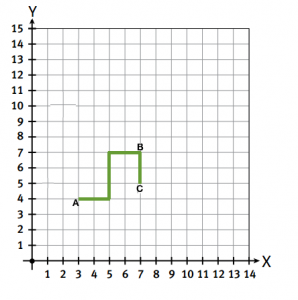# Minimum number of stops from given path

There are many points in two-dimensional space which need to be visited in a specific sequence. Path from one point to other is always chosen as shortest path and path segments are always aligned with grid lines. Now we are given the path which is chosen for visiting the points, we need to tell the minimum number of points that must be needed to generate given path.
Examples:

```In above diagram, we can see that there
must be at least 3 points to get above
path, which are denoted by A, B and C
```

## Recommended: Please try your approach on {IDE} first, before moving on to the solution.

We can solve this problem by observing the pattern of movement when visiting the stops. If we want to take the shortest path from one point to another point then we will move in either one or max two directions i.e. it is always possible to reach the other point following maximum two directions and if more than two directions are used then that path won’t be shortest, for example, path LLURD can be replaced with LLL only, so to find minimum number of stops in the path, we will loop over the characters of the path and maintain a map of directions taken till now. If at any index we found both ‘L’ as well as ‘R’ or we found both ‘U’ as well as ‘D’ then there must be a stop at current index, so we will increase the stop count by one and we will clear the map for next segment.

Total time complexity of the solution will be O(N)

 `// C++ program to find minimum number of points  ` `// in a given path ` `#include ` `using` `namespace` `std; ` ` `  `// method returns minimum number of points in given path ` `int` `numberOfPointInPath(string path) ` `{ ` `    ``int` `N = path.length(); ` ` `  `    ``// Map to store last occurrence of direction ` `    ``map<``char``, ``int``> dirMap; ` ` `  `    ``// variable to store count of points till now,  ` `    ``// initializing from 1 to count first point ` `    ``int` `points = 1; ` ` `  `    ``// looping over all characters of path string ` `    ``for` `(``int` `i = 0; i < N; i++) { ` ` `  `        ``// storing current direction in curDir  ` `        ``// variable ` `        ``char` `curDir = path[i]; ` ` `  `        ``// marking current direction as visited ` `        ``dirMap[curDir] = 1; ` ` `  `        ``// if at current index, we found both 'L' ` `        ``// and 'R' or 'U' and 'D' then current  ` `        ``// index must be a point ` `        ``if` `((dirMap[``'L'``] && dirMap[``'R'``]) ||  ` `            ``(dirMap[``'U'``] && dirMap[``'D'``])) { ` `             `  `            ``// clearing the map for next segment ` `            ``dirMap.clear(); ` ` `  `            ``// increasing point count ` `            ``points++; ` ` `  `            ``// revisitng current direction for next segment ` `            ``dirMap[curDir] = 1; ` `        ``} ` `    ``} ` ` `  `    ``// +1 to count the last point also ` `    ``return` `(points + 1); ` `} ` ` `  `// Driver code to test above methods ` `int` `main() ` `{ ` `    ``string path = ``"LLUUULLDD"``; ` `    ``cout << numberOfPointInPath(path) << endl; ` `    ``return` `0;  ` `} `

Output:

```3
```

This article is contributed by Utkarsh Trivedi. If you like GeeksforGeeks and would like to contribute, you can also write an article using contribute.geeksforgeeks.org or mail your article to contribute@geeksforgeeks.org. See your article appearing on the GeeksforGeeks main page and help other Geeks.

GeeksforGeeks has prepared a complete interview preparation course with premium videos, theory, practice problems, TA support and many more features. Please refer Placement 100 for details

My Personal Notes arrow_drop_up
Article Tags :
Practice Tags :

Be the First to upvote.

Please write to us at contribute@geeksforgeeks.org to report any issue with the above content.